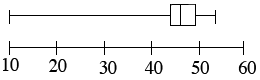### Home > MC2 > Chapter 6 > Lesson 6.1.3 > Problem6-31

6-31.

Here are the heights (in inches) of hollyhocks (tall, slender flowering plants) that are growing in the park: $10$, $39$, $43$, $45$, $46$$47$, $48$, $48$, $49$, $50$, and $52$. .

1. Find the median.

Since the data is already listed in ascending order, find the middle number.

$47$

2. Find the quartiles.

Find the median of the upper half and the lower half of the ordered set of data.

3. Make a box-and-whisker plot of the data.Use the eTool below to make a box plot.
Click the link at right for the full version of the eTool: MC2 6-31 HW eTool